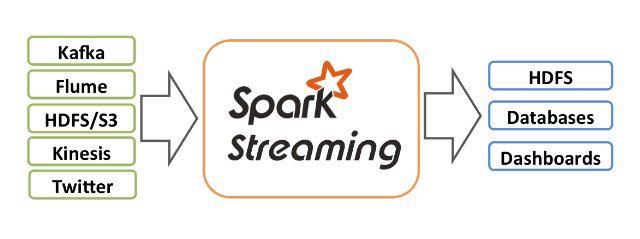## Wednesday, June 13, 2018

### Simple demo for spark streamingUSING PYSPARK

```[cloudera@quickstart ~]\$ pyspark
Python 2.6.6 (r266:84292, Jul 23 2015, 15:22:56)
```

2. Run Netcat
```[cloudera@quickstart ~]\$  nc -l localhost 2182
```

3. Type or paste the following code snippet
```from pyspark.streaming import StreamingContext

# Create a local StreamingContext with two working threads and a batch interval of 2 sec s```
```ssc = StreamingContext(sc, 2)

# Create a DStream
lines = ssc.socketTextStream("localhost", 2182)

# Split each line into words
words = lines.flatMap(lambda line: line.split(" "))

# Count each word in each batch
pairs = words.map(lambda word: (word, 1))
wordCounts = pairs.reduceByKey(lambda x, y: x + y)

# Print each batch
wordCounts.pprint()

ssc.start()             # Start the computation
ssc.awaitTermination()  # Wait for the computation to terminate
```

4. You should see lots of INFO interspersed with Timestamp corresponding to each batch that is updated every 2 seconds
```-------------------------------------------
Time: 2018-06-13 15:24:24
-------------------------------------------
```

5. When we type the following words in the netcat window we can see the output in the spark streaming job
```[cloudera@quickstart ~]\$  nc -l localhost 2182
hello world
hello hello hello
world world world
```

Output
```18/06/13 15:34:06 WARN storage.BlockManager:
Block input-0-1528929246600 replicated to only 0 peer(s) instead of 1 peers
-------------------------------------------
Time: 2018-06-13 15:34:08
-------------------------------------------
(u'hello', 3)
```

WITHOUT USING PYSPARK
• We can use any editor to create the spark application . In this example I am using vi editor
• Open the editor by specifying the name as shown below
```[cloudera@quickstart ~]\$  vi wordcount.py
```

Type or paste the following code snippet
```from pyspark import SparkContext
from pyspark.streaming import StreamingContext
# Create a local StreamingContext with two working threads and a batch interval of 2 sec s```
`sc  = SparkContext(appName="WordCount")`
```ssc = StreamingContext(sc, 2)

# Create a DStream
lines = ssc.socketTextStream("localhost", 2182)

# Split each line into words
words = lines.flatMap(lambda line: line.split(" "))

# Count each word in each batch
pairs = words.map(lambda word: (word, 1))
wordCounts = pairs.reduceByKey(lambda x, y: x + y)

# Print each batch
wordCounts.pprint()

ssc.start()             # Start the computation
ssc.awaitTermination()  # Wait for the computation to terminate
```

• We need to import sparkcontext and initialize sc
• Save the file with a name ex : wordcount.py
• Submit the spark application using spark-submit command
• Specify the hostname and the port in the spark-submit command
• Repeat step 4 and step 5

```[cloudera@quickstart ~]\$  spark-submit wordcount.py localhost 2182
```

import sys

from pyspark import SparkContext
from pyspark.streaming import StreamingContext

if __name__ == "__main__":

sc = SparkContext(appName="StreamingWordCount")
ssc = StreamingContext(sc, 30)

ssc.checkpoint("file:///tmp/spark")

lines = ssc.socketTextStream(sys.argv, int(sys.argv))

def countWords(newValues, lastSum):
if lastSum is None:
lastSum = 0
return sum(newValues, lastSum)

word_counts = lines.flatMap(lambda line: line.split(" "))\
.map(lambda word: (word, 1))\

word_counts.pprint()

ssc.start()
ssc.awaitTermination()

SUM THE INTEGERS RECEIVED IN A GIVEN INTERVAL

• Uses summary and inverse functions
• Sum the integers received in a certain window interval
```import sys
from pyspark import SparkContext
from pyspark.streaming import StreamingContext

if __name__ == "__main__":

sc = SparkContext(appName="StreamingWindowSum")

ssc = StreamingContext(sc, 2)

ssc.checkpoint("file:///tmp/spark")

lines = ssc.socketTextStream(sys.argv, int(sys.argv))

sum = lines.reduceByWindow(

lambda x, y: int(x) + int(y),
lambda x, y: int(x) - int(y),
10, 2)
sum.pprint()
ssc.start()
ssc.awaitTermination()
```